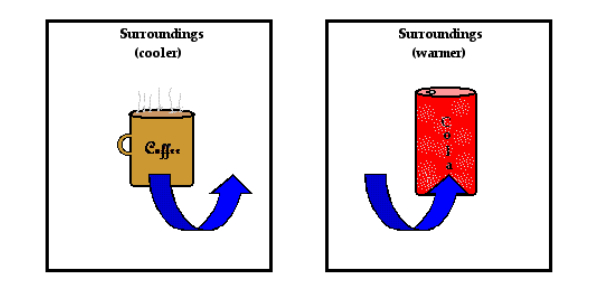# Heat Definition In Physics Quiz

10 Questions | Attempts: 756
ShareSettings.

• 1.
How much heat is given off when 27.0 g of steam condense to boiling water at 100°C?
• A.

61,020 J

• B.

9018 J

• C.

113 J

• D.

2,999 J

• 2.
How much heat is needed to warm 250.0 g of copper from 22° C to 98° C?
• A.

7315 J

• B.

2117 J

• C.

9433 J

• D.

79,420 J

• 3.
Which of the following is true when a substance is melting?
• A.

The temperature remains constant.

• B.

Both a solid and a gas can be present.

• C.

Heat is not required for the process since the temperature is constant.

• D.

The heat added is causing the particles to change from a high energy state to a low energy state.

• 4.
Is this equation an example of an exothermic or endothermic reaction: NaOH (s)  + H2O (l)  -->  NaOH (aq)  + 21 J
• A.

Exothermic

• B.

Endothermic

• C.

• D.

• 5.
If 900 J of heat are added to 100.0 g of water at 25.0 °C.  What is the final temperature of the water?
• A.

27.15 C

• B.

25.0 C

• C.

2.15 C

• D.

3.00 C

• 6.
What causes the process of perspiration to be cooling for  human skin?
• A.

It involves evaporation and is endothermic.

• B.

It involves evaporation and is exothermic.

• C.

It involves condensation and is endothermic.

• D.

It involves condensation.

• 7.
An 8.80 g sample of metal is heated to 92.0 °C and then  added to 14.77 g of water at 20.0 °C in an insulated  calorimeter. At thermal equilibrium the temperature of the  system was measured as 25.0 °C. What is the identity of the  metal?
• A.

Titanium

• B.

• C.

Copper

• D.

Tin

• 8.
Which best explains the relationship between heat energy and temperature?
• A.

As heat energy decreases and temperature remains constant, condensation occurs.

• B.

As heat energy increases and temperature increases, freezing occurs.

• C.

As heat energy decreases and temperature remains constant, evaporation occurs.

• D.

As heat energy increases and temperature decreases, melting occurs.

• 9.
Why does it require 5,511 J of heat energy to melt 16.5 g of ice?
• A.

334 J/g of heat energy is absorbed by the ice as it is converted from a solid to a liquid.

• B.

2,260 J/g of heat energy is absorbed by the ice as it is converted from a solid to a liquid.

• C.

4.18 J/g°C of heat energy is required as ice is converted from a solid to a liquid.

• D.

2.05 J/g°C of heat energy is required as ice is converted from a solid to a liquid.

• 10.
The graph below below shows a substance heating from 40 C to 140 C.  If 70 kJ of heat are removed from the substance when it is at 140°C, what will be the state and temperature of the substance?
• A.

Liquid at 60 C.

• B.

Liquid at 10 C.

• C.

Gas at 100 C.

• D.

Gas at 120 C.

## Related TopicsBack to top
×

Wait!
Here's an interesting quiz for you.